# 2. 共表达网络构建及模块获取

• 自动法：采用自动的网络构建和模块检测功能，快速获得结果
• 逐步法：采用分布的网络构建和模块检测功能，以便使用者在每一步中可以选择自定义的方法或者可替代的方法
• 分块法：一个自动的分块的网络建设和模块检测方法，解决分析数据集太大，不能在一次运行的情况

1. 如果不像太折腾，就使用自动法，快速构建共表达网络并识别模块
2. 如果需要根据自己数据集的特征调整参数，要么调整自动法的函数的参数，或者采用逐步法，在每一步的处理中设置合适的参数
3. 在遇到要处理的基因太多的时候，就采用分块法，这是在自动法的基础上，先将数据集分成几块，然后分别计算，并在最后合并。

## 2.1. 导入处理的数据：

``````# 设定工作目录
setwd("/home/cxt/data/R/")
# 导入包
library(WGCNA)
# 设置将strings不要转成factors
options(stringsAsFactors=F)

# 允许使用最大线程
# 或者直接指定线程数

# 导入数据
lnames
``````

## 2.2. 自动法：网络构建和模块检测

### 2.2.1 确定软阈值β

``````# 软阈值的预设范围
powers <- c(c(1:10), seq(from=12, to=20, by=2))
# 自动计算推荐的软阈值
sft <- pickSoftThreshold(datExpr, powerVector=powers, verbose=5, networkType="unsigned")
# 推荐值。如果是NA，就需要画图来自己挑选
sft\$powerEstimate

# 作图
sizeGrWindow(9, 5)
par(mfrow=c(1, 2))
cex1 <- 0.9

# Scale-free topology fit index 作为评价软阈值指标
plot(sft\$fitIndices[, 1], -sign(sft\$fitIndices[, 3])*sft\$fitIndices[, 2],
xlab="Soft Threshold (power)", ylab="Scale Free Topology Model Fit,signed R^2", type="n",
main=paste("Scale independence"))
text(sft\$fitIndices[, 1], -sign(sft\$fitIndices[, 3])*sft\$fitIndices[, 2],
labels=powers, cex=cex1, col="red")
# h值作为筛选指标
abline(h=0.80, col="red")

# Mean connectivity 作为评价软阈值的指标
plot(sft\$fitIndices[, 1], sft\$fitIndices[, 5],
xlab="Soft Threshold (power)", ylab="Mean Connectivity", type="n",
main=paste("Mean connectivity"))
text(sft\$fitIndices[, 1], sft\$fitIndices[, 5], labels=powers, cex=cex1, col="red")

# 如果是NA，此时就需要根据图来自己指定数值了
sft\$powerEstimate <- 6
``````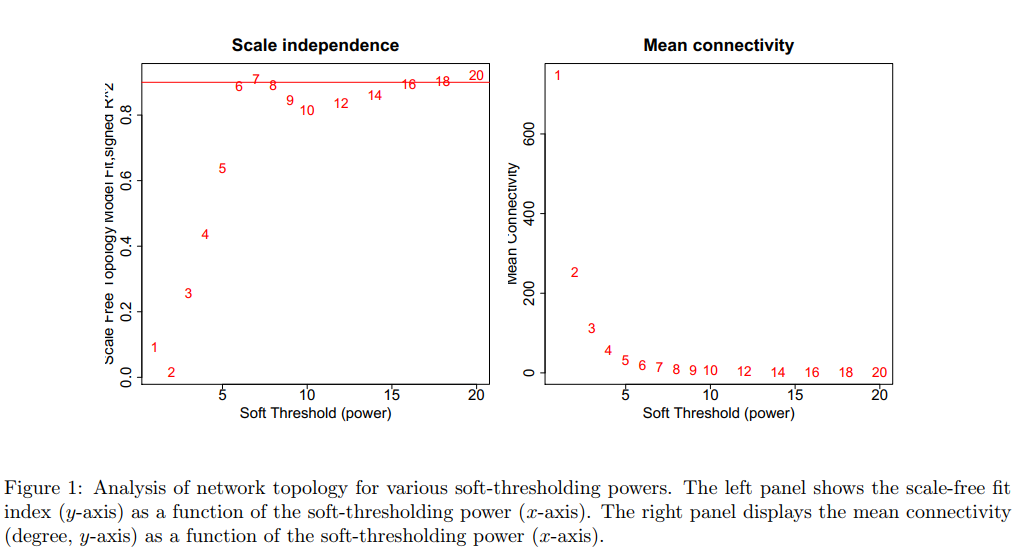### 2.2.2 构建网络并识别模块

``````# 构建网络
# deepSplit是生成模块的梯度，从[0:4]中选择，越大模块越多
# minModuleSize最小模块的基因数目
# mergeCutHeight是合并相似的模块的合并系数，是通过主成分分析出来的
# mumericLabels 已数字命名模块
net <- blockwiseModules(datExpr, power = sft\$powerEstimate,
TOMType = "unsigned", minModuleSize = 30,
reassignThreshold = 0, mergeCutHeight = 0.25,
numericLabels = TRUE, pamRespectsDendro = FALSE,
saveTOMs = TRUE,
saveTOMFileBase = "femaleMouseTOM",
verbose = 3)

# 查看每个模块的基因数，其中0模块下为没有计算进入模块的基因数
table(net\$colors)
``````

### 2.2.3. 结果可视化

``````# 打开新的绘图窗口
sizeGrWindow(12, 9)
# 把模块编号转成颜色
mergedColors <- labels2colors(net\$colors)
# 绘制树状图和下面的模块颜色
plotDendroAndColors(net\$dendrograms[], mergedColors[net\$blockGenes[]],
"Module colors",
dendroLabels = FALSE, hang = 0.03,
addGuide = TRUE, guideHang = 0.05)
``````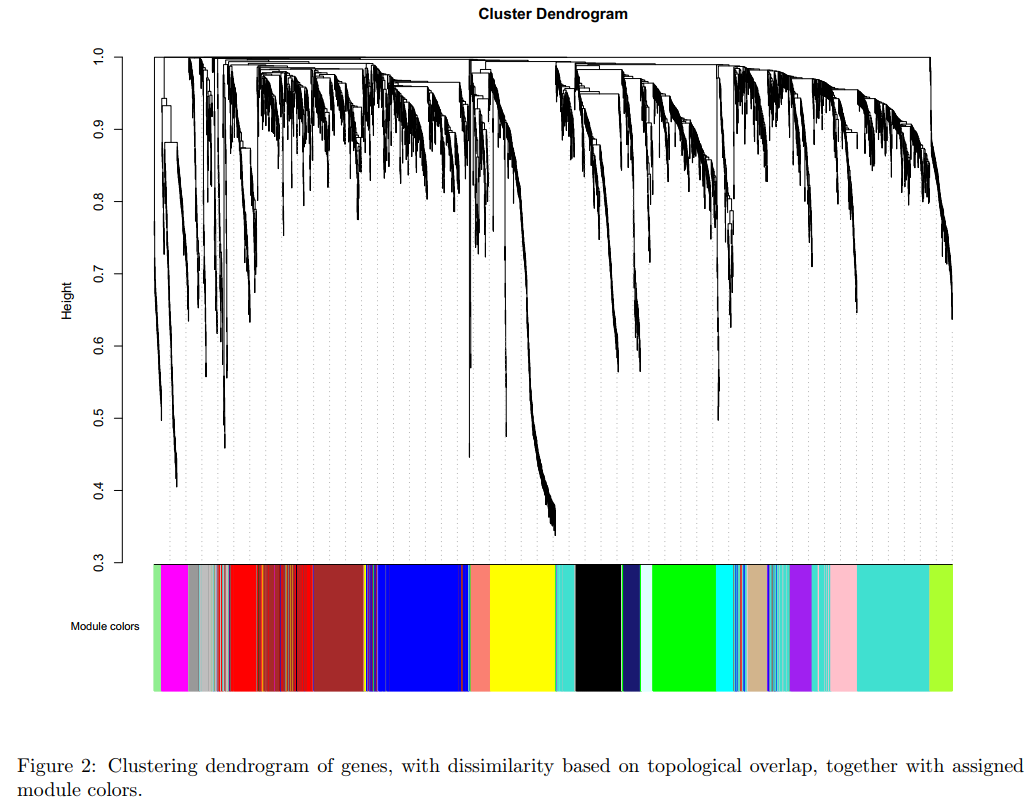### 2.2.4. 保存结果

``````# 计算模块特征向量MEs
moduleLabels <- net\$colors
moduleColors <- labels2colors(net\$colors)
MEs <- net\$MEs
geneTree <- net\$dendrograms[]
# 保存数据
save(MEs, moduleLabels, moduleColors, geneTree,
file="networkConstruction-auto.RData")
``````

## 2.3. 逐步法：网络构建和模块检测

### 2.3.1. 确定软阈值β

`2.2.1 确定软阈值β`相同

### 2.3.2. 计算共表达相似性和邻接矩阵

``````# 软阈值β为sft\$powerEstimate
``````

### 2.3.3. 构建拓扑重叠矩阵

``````# Turn adjacency into topological overlap
dissTOM = 1-TOM
``````

### 2.3.4. 基因聚类分析

``````# 调用层次聚类函数
geneTree = hclust(as.dist(dissTOM), method = "average");
# 画出层次聚类树
sizeGrWindow(12,9)
plot(geneTree, xlab="", sub="", main = "Gene clustering on TOM-based dissimilarity",
labels = FALSE, hang = 0.04);
``````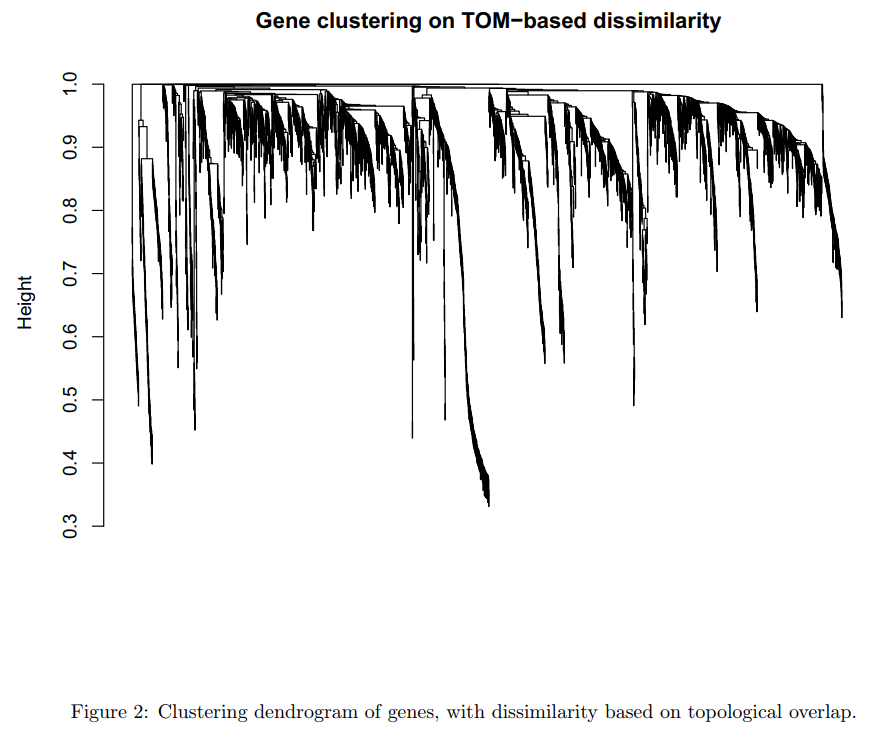### 2.3.5. 模块识别

``````# 如果想要大的模块，就把最小模块的的大小设置的高一点
minModuleSize = 30;
# 使用动态树剪切（Dynamic Tree Cut）确定模块
dynamicMods = cutreeDynamic(dendro = geneTree, distM = dissTOM,
deepSplit = 2, pamRespectsDendro = FALSE,
minClusterSize = minModuleSize);
table(dynamicMods)
``````

``````# 将模块的数字标签转换为颜色标签
dynamicColors = labels2colors(dynamicMods)
table(dynamicColors)
# 绘制聚类树及其模块
sizeGrWindow(8,6)
plotDendroAndColors(geneTree, dynamicColors, "Dynamic Tree Cut",
dendroLabels = FALSE, hang = 0.03,
addGuide = TRUE, guideHang = 0.05,
main = "Gene dendrogram and module colors")
``````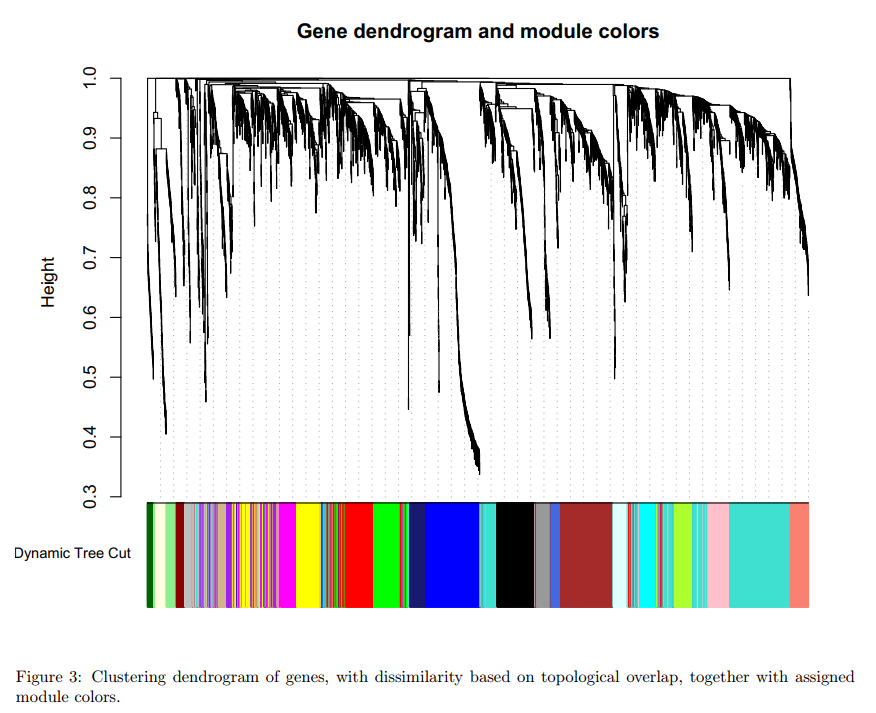### 3.4.6. 模块合并

``````# 计算模块特征基因
MEList = moduleEigengenes(datExpr, colors = dynamicColors)
MEs = MEList\$eigengenes
# 计算模块特征基因的不同
MEDiss = 1-cor(MEs);
# 聚类模块特征基因
METree = hclust(as.dist(MEDiss), method = "average");
# 绘制结果
sizeGrWindow(7, 6)
plot(METree, main = "Clustering of module eigengenes",
xlab = "", sub = "")
``````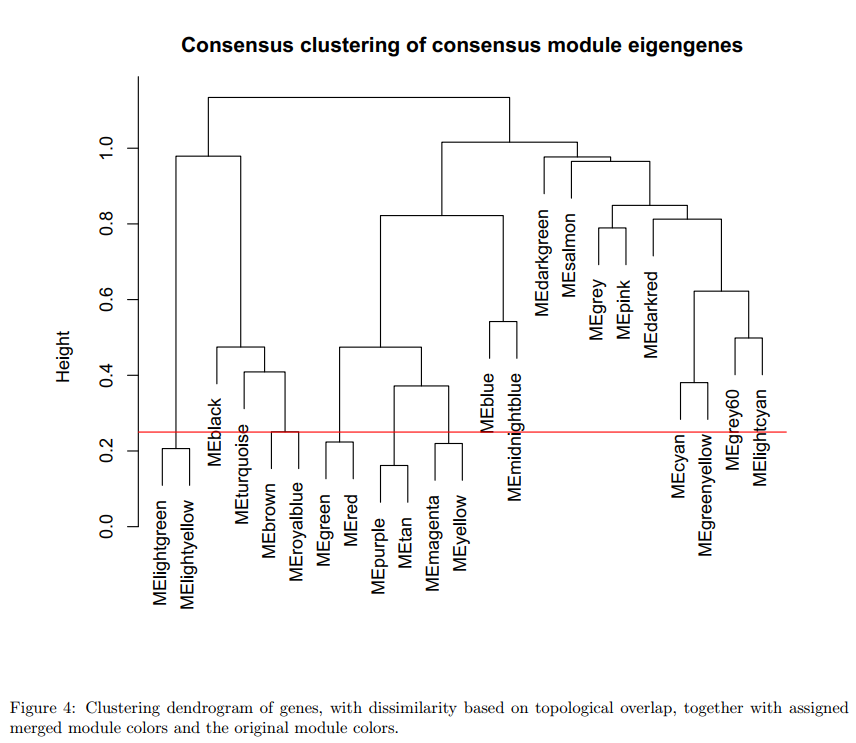``````MEDissThres = 0.25
# 在聚类树中绘制切线
abline(h=MEDissThres, col = "red")
# 使用一个自动合并函数
merge = mergeCloseModules(datExpr, dynamicColors, cutHeight = MEDissThres, verbose = 3)
# 合并模块的颜色
mergedColors = merge\$colors;
# 新的合并模块的特征基因
mergedMEs = merge\$newMEs;
``````

``````sizeGrWindow(12, 9)
#pdf(file = "Plots/geneDendro-3.pdf", wi = 9, he = 6)
plotDendroAndColors(geneTree, cbind(dynamicColors, mergedColors),
c("Dynamic Tree Cut", "Merged dynamic"),
dendroLabels = FALSE, hang = 0.03,
addGuide = TRUE, guideHang = 0.05)
#dev.off()
``````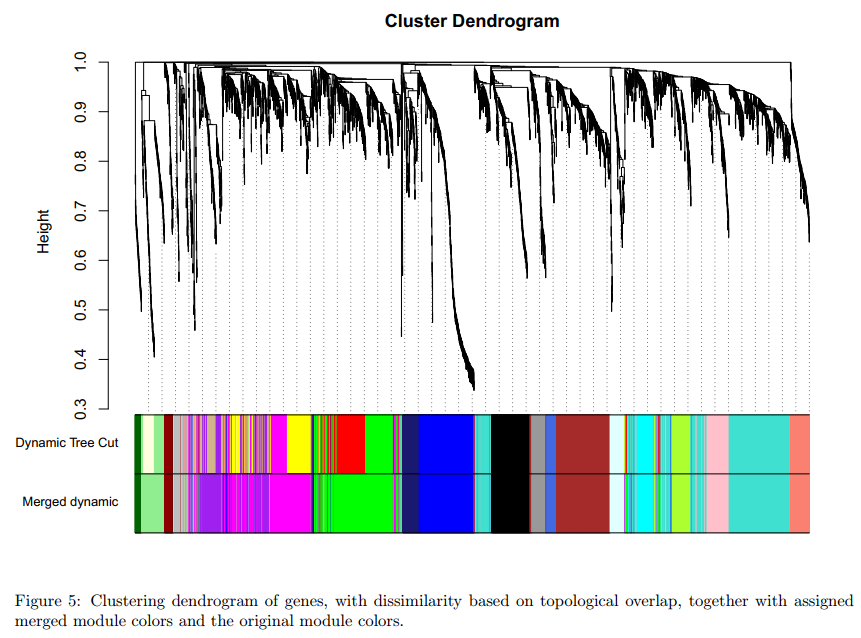### 3.4.7. 保存数据

``````# moduleColors重命名为合并模块的颜色名称
moduleColors = mergedColors
# 构造与颜色相对应的数字标签
colorOrder = c("grey", standardColors(50));
moduleLabels = match(moduleColors, colorOrder)-1;
MEs = mergedMEs;
# 保存模块颜色和标签，以供后续部分使用
save(MEs, moduleLabels, moduleColors, geneTree, file = "networkConstruction-stepByStep.RData")
``````

## 2.4. 分块法：网络构建和模块检测

### 2.4.1. 确定软阈值β

`2.2.1 确定软阈值β`相同

### 2.4.2. 分块构建网络并识别模块

``````bwnet = blockwiseModules(datExpr, maxBlockSize = 2000,
power = sft\$powerEstimate, TOMType = "unsigned",
minModuleSize = 30,
reassignThreshold = 0, mergeCutHeight = 0.25,
numericLabels = TRUE,
saveTOMs = TRUE,
saveTOMFileBase = "femaleMouseTOM-blockwise",
verbose = 3)
``````

1. 函数`blockwiseModules`有许多参数，目前提供的参数可能不适合用户希望分析的特定数据集，对于想要根据自己的数据调整代码的，可以阅读R环境中包中提供的帮助文件，并尝试调整网络结构和模块检测参数，以获得更好的（生物学上更相关）分析结果。
2. 关于块大小的第二个警告。特别是，参数`maxBlockSize`告诉函数读者计算机可以处理的最大块的大小。在这个例子中，我们将最大块大小设置为2000来说明块分析及其结果，但对于大多数现代计算机来说，这个值是不必要的小。默认值为5000，适用于大多数现代桌面。如果服务器可以访问内存超过4 GB的大型工作站，则可以增加参数`maxBlockSize`。 1）16GB工作站最多可处理20000个探测器；2）32GB工作站应该可以处理30000个；3）4GB标准台式机或笔记本电脑可以处理多达8000-10000个探测器。通常，最好以尽可能少的块分析数据集。

### 2.4.3. 结果比较：与自动法

#### 2.4.3.1. 导入自动法分析的结果

``````# 导入自动法最终保存的结果
# 模块标签重命名
bwLabels = matchLabels(bwnet\$colors, moduleLabels);
# 将标签转换为用于绘图的颜色
bwModuleColors = labels2colors(bwLabels)
# 查看模块信息：
table(bwLabels)
0 1 2 3 4 5 6 7 8 9 10 11 12 13 14 15 16 17 18 19 20
142 472 470 479 271 327 130 209 153 121 100 100 104 77 73 81 40 42 34 91 84
# 表示有20个模块，标记为1到20，标记为0的是所有模块外的基因。
``````

#### 2.4.3.2. 绘制分块法获得的结果

``````# 打开新绘图窗口
sizeGrWindow(6,6)
# 绘制block 1中的聚类树和模块
plotDendroAndColors(bwnet\$dendrograms[], bwModuleColors[bwnet\$blockGenes[]],
"Module colors", main = "Gene dendrogram and module colors in block 1",
dendroLabels = FALSE, hang = 0.03,
addGuide = TRUE, guideHang = 0.05)
# 绘制block 2中的聚类树和模块
plotDendroAndColors(bwnet\$dendrograms[], bwModuleColors[bwnet\$blockGenes[]],
"Module colors", main = "Gene dendrogram and module colors in block 2",
dendroLabels = FALSE, hang = 0.03,
addGuide = TRUE, guideHang = 0.05)
``````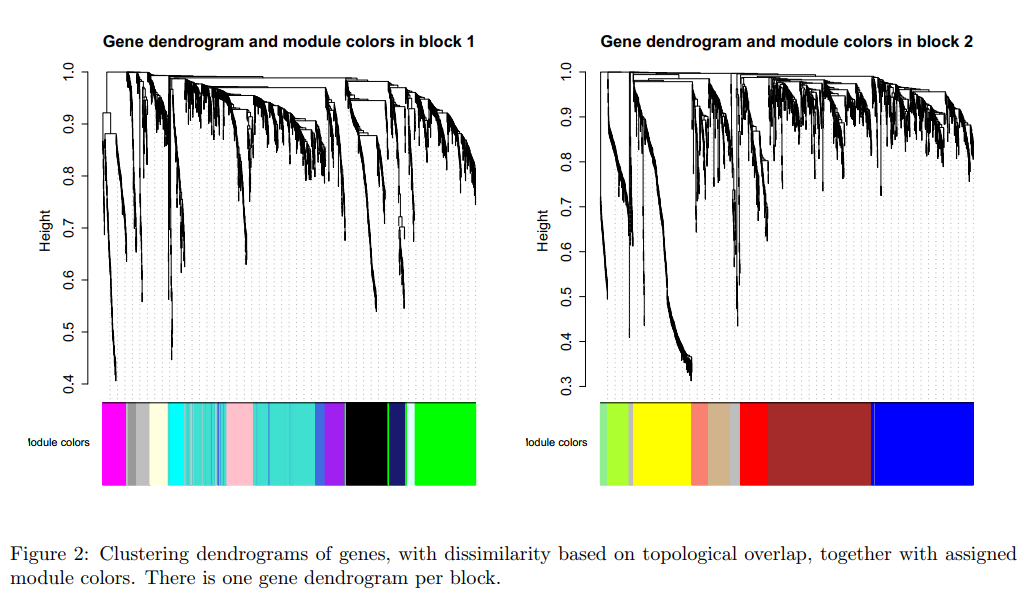#### 2.4.3.3. 比较分块法和自动法结果

1）目视检查

``````sizeGrWindow(12,9)
plotDendroAndColors(geneTree,
cbind(moduleColors, bwModuleColors),
c("Single block", "2 blocks"),
main = "Single block gene dendrogram and module colors",
dendroLabels = FALSE, hang = 0.03,
addGuide = TRUE, guideHang = 0.05)
``````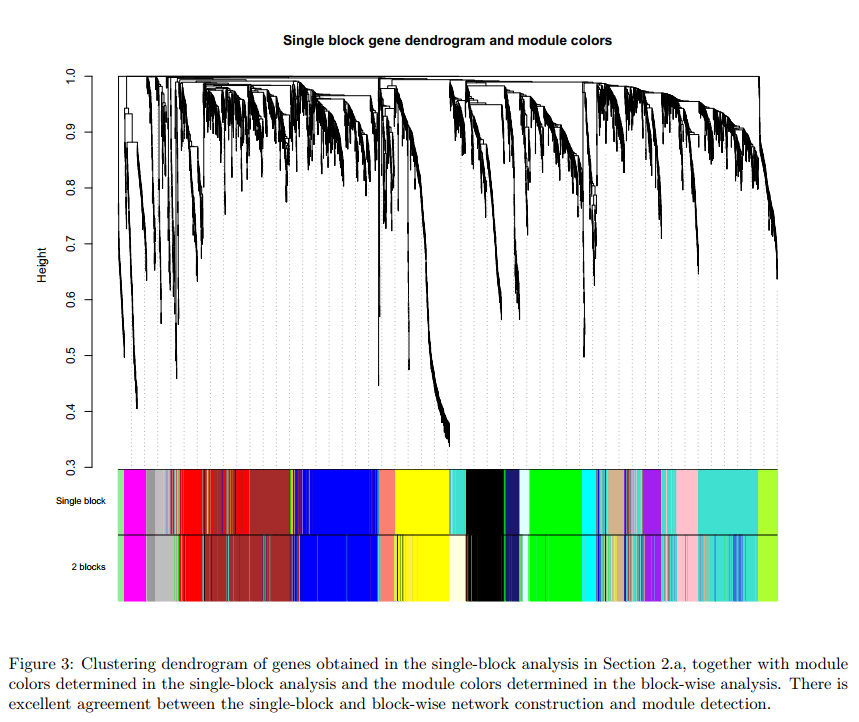**结果：**目视检查证实，在分块法和自动法识别的模块有很好的一致性

2）模块特征基因比较

``````# 自动法：模块特征基因
singleBlockMEs = moduleEigengenes(datExpr, moduleColors)\$eigengenes;
# 分块法：模块特征基因
blockwiseMEs = moduleEigengenes(datExpr, bwModuleColors)\$eigengenes;
``````

``````# 匹配两种方法的模块
single2blockwise = match(names(singleBlockMEs), names(blockwiseMEs))
# 比较模块中的特征基因相关性
signif(diag(cor(blockwiseMEs[, single2blockwise], singleBlockMEs)), 3)
``````

### 评论

###### Your browser is out of date!

Update your browser to view this website correctly. Update my browser now

×